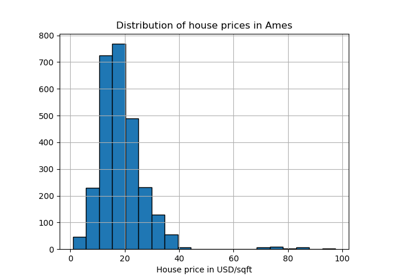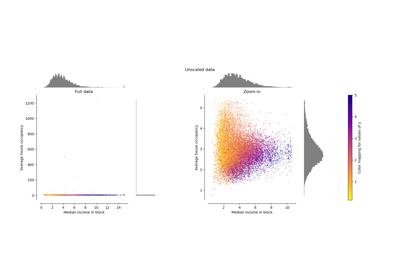# sklearn.preprocessing.RobustScaler¶

class sklearn.preprocessing.RobustScaler(*, with_centering=True, with_scaling=True, quantile_range=(25.0, 75.0), copy=True, unit_variance=False)[source]

Scale features using statistics that are robust to outliers.

This Scaler removes the median and scales the data according to the quantile range (defaults to IQR: Interquartile Range). The IQR is the range between the 1st quartile (25th quantile) and the 3rd quartile (75th quantile).

Centering and scaling happen independently on each feature by computing the relevant statistics on the samples in the training set. Median and interquartile range are then stored to be used on later data using the transform method.

Standardization of a dataset is a common preprocessing for many machine learning estimators. Typically this is done by removing the mean and scaling to unit variance. However, outliers can often influence the sample mean / variance in a negative way. In such cases, using the median and the interquartile range often give better results. For an example visualization and comparison to other scalers, refer to Compare RobustScaler with other scalers.

New in version 0.17.

Read more in the User Guide.

Parameters:
with_centeringbool, default=True

If True, center the data before scaling. This will cause transform to raise an exception when attempted on sparse matrices, because centering them entails building a dense matrix which in common use cases is likely to be too large to fit in memory.

with_scalingbool, default=True

If True, scale the data to interquartile range.

quantile_rangetuple (q_min, q_max), 0.0 < q_min < q_max < 100.0, default=(25.0, 75.0)

Quantile range used to calculate scale_. By default this is equal to the IQR, i.e., q_min is the first quantile and q_max is the third quantile.

New in version 0.18.

copybool, default=True

If False, try to avoid a copy and do inplace scaling instead. This is not guaranteed to always work inplace; e.g. if the data is not a NumPy array or scipy.sparse CSR matrix, a copy may still be returned.

unit_variancebool, default=False

If True, scale data so that normally distributed features have a variance of 1. In general, if the difference between the x-values of q_max and q_min for a standard normal distribution is greater than 1, the dataset will be scaled down. If less than 1, the dataset will be scaled up.

New in version 0.24.

Attributes:
center_array of floats

The median value for each feature in the training set.

scale_array of floats

The (scaled) interquartile range for each feature in the training set.

New in version 0.17: scale_ attribute.

n_features_in_int

Number of features seen during fit.

New in version 0.24.

feature_names_in_ndarray of shape (n_features_in_,)

Names of features seen during fit. Defined only when X has feature names that are all strings.

New in version 1.0.

robust_scale

Equivalent function without the estimator API.

sklearn.decomposition.PCA

Further removes the linear correlation across features with ‘whiten=True’.

Notes

Examples

>>> from sklearn.preprocessing import RobustScaler
>>> X = [[ 1., -2.,  2.],
...      [ -2.,  1.,  3.],
...      [ 4.,  1., -2.]]
>>> transformer = RobustScaler().fit(X)
>>> transformer
RobustScaler()
>>> transformer.transform(X)
array([[ 0. , -2. ,  0. ],
[-1. ,  0. ,  0.4],
[ 1. ,  0. , -1.6]])


Methods

 fit(X[, y]) Compute the median and quantiles to be used for scaling. fit_transform(X[, y]) Fit to data, then transform it. get_feature_names_out([input_features]) Get output feature names for transformation. Get metadata routing of this object. get_params([deep]) Get parameters for this estimator. Scale back the data to the original representation. set_output(*[, transform]) Set output container. set_params(**params) Set the parameters of this estimator. Center and scale the data.
fit(X, y=None)[source]

Compute the median and quantiles to be used for scaling.

Parameters:
X{array-like, sparse matrix} of shape (n_samples, n_features)

The data used to compute the median and quantiles used for later scaling along the features axis.

yIgnored

Not used, present here for API consistency by convention.

Returns:
selfobject

Fitted scaler.

fit_transform(X, y=None, **fit_params)[source]

Fit to data, then transform it.

Fits transformer to X and y with optional parameters fit_params and returns a transformed version of X.

Parameters:
Xarray-like of shape (n_samples, n_features)

Input samples.

yarray-like of shape (n_samples,) or (n_samples, n_outputs), default=None

Target values (None for unsupervised transformations).

**fit_paramsdict

Returns:
X_newndarray array of shape (n_samples, n_features_new)

Transformed array.

get_feature_names_out(input_features=None)[source]

Get output feature names for transformation.

Parameters:
input_featuresarray-like of str or None, default=None

Input features.

• If input_features is None, then feature_names_in_ is used as feature names in. If feature_names_in_ is not defined, then the following input feature names are generated: ["x0", "x1", ..., "x(n_features_in_ - 1)"].

• If input_features is an array-like, then input_features must match feature_names_in_ if feature_names_in_ is defined.

Returns:
feature_names_outndarray of str objects

Same as input features.

Get metadata routing of this object.

Please check User Guide on how the routing mechanism works.

Returns:

A MetadataRequest encapsulating routing information.

get_params(deep=True)[source]

Get parameters for this estimator.

Parameters:
deepbool, default=True

If True, will return the parameters for this estimator and contained subobjects that are estimators.

Returns:
paramsdict

Parameter names mapped to their values.

inverse_transform(X)[source]

Scale back the data to the original representation.

Parameters:
X{array-like, sparse matrix} of shape (n_samples, n_features)

The rescaled data to be transformed back.

Returns:
X_tr{ndarray, sparse matrix} of shape (n_samples, n_features)

Transformed array.

set_output(*, transform=None)[source]

Set output container.

See Introducing the set_output API for an example on how to use the API.

Parameters:
transform{“default”, “pandas”}, default=None

Configure output of transform and fit_transform.

• "default": Default output format of a transformer

• "pandas": DataFrame output

• None: Transform configuration is unchanged

Returns:
selfestimator instance

Estimator instance.

set_params(**params)[source]

Set the parameters of this estimator.

The method works on simple estimators as well as on nested objects (such as Pipeline). The latter have parameters of the form <component>__<parameter> so that it’s possible to update each component of a nested object.

Parameters:
**paramsdict

Estimator parameters.

Returns:
selfestimator instance

Estimator instance.

transform(X)[source]

Center and scale the data.

Parameters:
X{array-like, sparse matrix} of shape (n_samples, n_features)

The data used to scale along the specified axis.

Returns:
X_tr{ndarray, sparse matrix} of shape (n_samples, n_features)

Transformed array.

## Examples using sklearn.preprocessing.RobustScaler¶Evaluation of outlier detection estimators

Evaluation of outlier detection estimatorsCompare the effect of different scalers on data with outliers

Compare the effect of different scalers on data with outliers